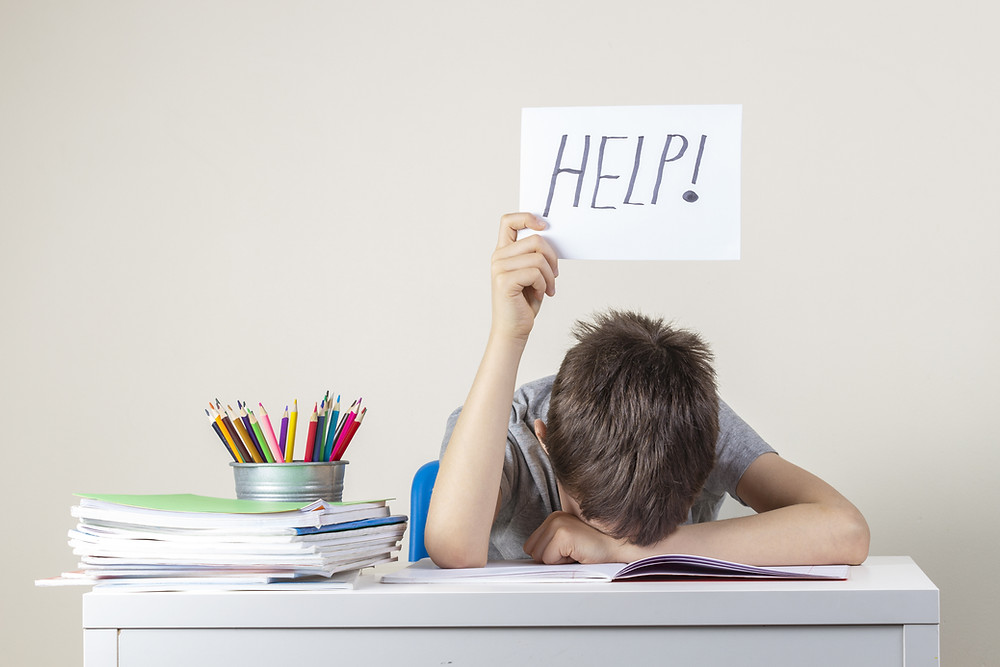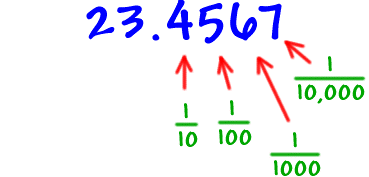Search
• by Blaine Helwig

# Teaching Decimal Place Value and Value – Use the PointA frustrating math moment I remember as a ten year old is decimal numbers and understanding both place value and value of the digits (numbers) to the right of the decimal point. I vividly recall sitting at my kitchen table trying to understand why the first place value location after the decimal point was not the ‘oneths’ place --- but the tenths. Whole number place value begins with the ones, tens, hundreds and so forth as we add digits to the left, then why did the decimal digits not symmetrically repeat to the right of the decimal point?

Today, regardless of the school’s socioeconomic setting students often demonstrate the same decimal difficulty with decimal place value and value - since it is a difficult arithmetic math topic. A physical understanding of digits (numbers) to the right of the decimal point is difficult with only one or two numbers, but the fact that each digit added after the decimal point is ten times smaller than the preceding digit often seems counter intuitive to kids. Frequently, the core lesson evolves into a pedagogical wheel of repetition until kids submit and memorize both the place value and the value of each digit to the right of the decimal – unsure of number sense of digits or mathematical significance of the decimal.As I sat at my kitchen table, struggling with decimal place value, my father noticed that I was consistently answering the decimal homework problems incorrectly. In thirty seconds, he taught me a method to understand both decimal place value and value that I used successfully with my own students.

Understanding decimal place value and value methodology

Place a ‘1’ directly underneath the decimal point. Add a zero under every digit (including a zero digit) to the right of the decimal point. Hence, each digit may be read individually with regard to its place value: tenths, hundredths, thousandths, etc.

For example:

Given the decimal: 6.204 6.204

1000

After adding the ‘1’ and three (3) zeros to match each digit in the decimal to the right of the decimal point, the original decimal is transformed to an equivalent mixed number (with a decimal point, of course).

Students readily understand the following about the decimal moving from the decimal point to the right reading the decimal one digit at time:

• the ‘2’ digit is in the ‘tenths’ place and has a value of 2/10’s;

• the ‘0’ digit is in the ‘hundredths’ place and has a value of 0/100's;

• the ‘4’ is in the ‘thousandths’ place and has a value of 4/1,000's;

• the 'whole' proper fraction to the right of the decimal point is 204/1,000's.

• And, based on money (magnitude - explained below), it is about \$6.20.

Importantly, it also relates the decimal digit(s) to a proper fraction that lends a physical understanding of each digit’s magnitude/size in the decimal number. Students can also visually comprehend that as digits (i.e. numbers) are added to the right of the decimal, each digit is ten times smaller than the preceding digit. It is also recommended that classroom teacher not only stress each individual digit in the decimal, but all digits to the right of the decimal (i.e. 204) to illustrate the magnitude of the decimal. For example, the decimal 6.204; there are 204 out of 1,000 squares shaded, so the decimal 6.204 is closer to the whole number 6 than it is to 7.Finally, most students possess the most decimal background knowledge in terms of money – with only two digits behind the decimal point. Another way to effectively illustrate the ‘size’ of a decimal is by circling only two places to the right of the decimal point (i.e. to the hundredths place).

For example, the decimal 2.08407 has a magnitude or size of about 2.08. The other 3 digits (407) do not significantly affect the overall size or magnitude of the decimal. The student realizes that the decimal 2.08407 is between the whole numbers 2 and 3, and the decimal is closer to 2 than 3 by 0.08 or 8/100’s. Repeat daily until mastered, but include the decimal and fractional distances to 2.5 and 3.0, in this example. This methodology takes the mystery of decimals for elementary and middle school students. Decimals become manageable mathematics for students very rapidly.